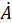Daily Practice Problems
Class 11 Physics
Kinetic TheoryQuestion 1.

What do you understand by ‘Mean free path’?

Question 2.

A nitrogen container of volume 45 litres has an initial gauge pressure of 20 atm and a temperature of 30 °C. After some nitrogen is withdrawn from the container, the gauge pressure drops to 15 atm and its temperature drops to 15 °C. Estimate the mass of nitrogen taken out of the container (R = 8.31 J mol–1 K–1, molecular mass of N2 = 28 u).

Question 3.

Question 4.

A vessel contains two non-reactive gases: neon (monatomic) and oxygen (diatomic). The ratio of their partial pressures is 5:3. Estimate the ratio of (i) number of molecules and (ii) mass density of neon and oxygen in the vessel. Atomic mass of Ne = 20.2 u, molecular mass of O2 = 32.0 u.

Question 5.

Give ideal gas equation.

Question 6.

A flask contains argon and chlorine in the ratio of 3:2 by mass. The temperature of the mixture is 25 °C. Obtain the ratio of (i) average kinetic energy per molecule, and (ii) root mean square speed vrms of the molecules of the two gases. Atomic mass of argon = 39.9 u; Molecular mass of chlorine = 70.9 u.

Question 7.

An air bubble of volume 1.5 cm3 rises from the bottom of a lake 50 m deep at a temperature of 15 °C. To what volume does it grow when it reaches the surface, which is at a temperature of 30 °C?

Question 8.

What do you mean by kinetic interpretation of temperature?

Question 9.

What is the relation between specific heat capacities of monatomic gases?

Question 10.

Write down the specific heat capacities for diatomic gases.

Question 11.

A cylinder of fixed capacity 44.8 litres contains helium gas at standard temperature and pressure. What is the amount of heat needed to raise the temperature of the gas in the cylinder by 25.0 °C? (R = 8.31 J mol–1 K–1).

Question 12.

What do law of equipartition of energy states?

Question 13.

What is the ratio of cp and cv for polyatomic gases?

Question 14.

Give formula for specific heat capacity for solids.

Question 15.

A metre long narrow bore held horizontally (and closed at one end) contains a 80 cm long mercury thread, which traps a 12 cm column of air. What happens if the tube is held vertically with the open end at the bottom?

Question 16.

Estimate the mean free path and collision frequency of an oxygen molecule in a cylinder containing oxygen at 3.0 atm and temperature 150C. Take the radius of an oxygen molecule to be roughly 1.05 Å. Compare the collision time with the time the molecule moves freely between two successive collisions (Molecular mass of O2 = 32.0 u).

Question 17.

Estimate the average thermal energy of a helium atom at the temperature 40 °C.

Question 18.

Calculate the mean free path of a molecule at 250C, if the distance covered in two successive collisions is 1.0Aand the pressure inside the container is 3.0 atm.

Question 19.

Estimate the total number of air molecules (inclusive of oxygen, nitrogen, water vapour and other constituents) in a room of capacity 30.0 m3 at a temperature of 25°C and 1 atm pressure.

Question 20.

At what temperature is the root mean square speed of an atom in an argon gas cylinder equal to the rms speed of a helium gas atom at – 10 °C? (Atomic mass of Ar = 39.9 u, of He = 4.0 u).

**********